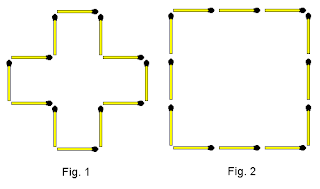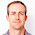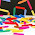## 31 December 2013

### 121. Create 3 square units with 12 sticks.

 Easy Medium Hard ExtremeAssume the length of one matchstick is 1 unit.

In figure 1 we used 12 sticks to form an area of 5 square units. In figure 2 we also used 12 sticks, but this time the area is 9 square units.

How would you create an area of 3 square units using 12 sticks and not breaking any of the sticks? This one is not so easy...!
The total area of the triangle ABC:
= 1/2 X BC X AB
= 1/2 X 3 X 4
= 6 square units

The area marked "D" can easily be calculated as 3 square units.

Therefore the area marked "E":
= Area ABC - Area D
= 6 - 3
= 3 square units

Any other ideas?

Hide Answer

#### 4 comments:

1.Place three 1X1 squares one at others corner...

|_|
|_|
|_|

close the lids... :)

2._ _
|_|_|_|
|_|

3.|V|
| |
| |
| |
V

This shape when the distance between the parallel sides is 3/4 unit.

1.Great - hope to include answer soon!